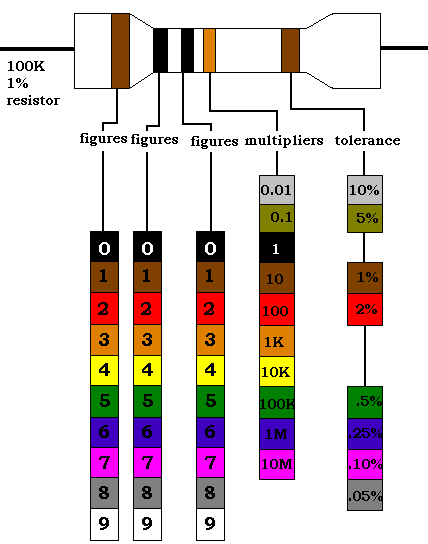# How to Read Resistor Codes

Here is the diagram that should be used to decipher the resistor codes:First find the tolerance band, it will typically be gold ( 5%) and sometimes silver (10%).

Starting from the other end, identify the first band - write down the number associated with that color; in this case Blue is 6.

Now 'read' the next color, here it is red so write down a '2' next to the six. (you should have '62' so far.)

Now read the third or 'multiplier' band and write down that number of zeros.

In this example it is two so we get '6200' or '6,200'. If the 'multiplier' band is Black (for zero) don't write any zeros down.

If the 'multiplier' band is Gold move the decimal point one to the left. If the 'multiplier' band is Silver move the decimal point two places to the left. If the resistor has one more band past the tolerance band it is a quality band.

Read the number as the '% Failure rate per 1000 hour' This is rated assuming full wattage being applied to the resistors. (To get better failure rates, resistors are typically specified to have twice the needed wattage dissipation that the circuit produces) 1% resistors have three bands to read digits to the left of the multiplier. They have a different temperature coefficient in order to provide the 1% tolerance.

At 1% - the temperature coefficient starts to become an important factor. At +/-200 ppm a change in temperature of 25 Deg C causes a value change of up to 1%.